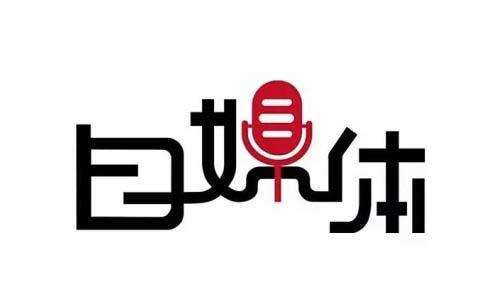免费咨询热线：023-6702-6306

想要自媒体营销推广做得好，软文很重要！1、新闻媒体力量不可或缺

2、社交平台推广增加互动

3、利用营销机构来实现全网同步发布

<<<<<<<<<<<<<<<<<<<<<<<<<<<<<<<<<<<<<<<<<<<<<<<<<<<<<<<<<<<<<<<<<<<<<<<<<<<<<<<<<<<<